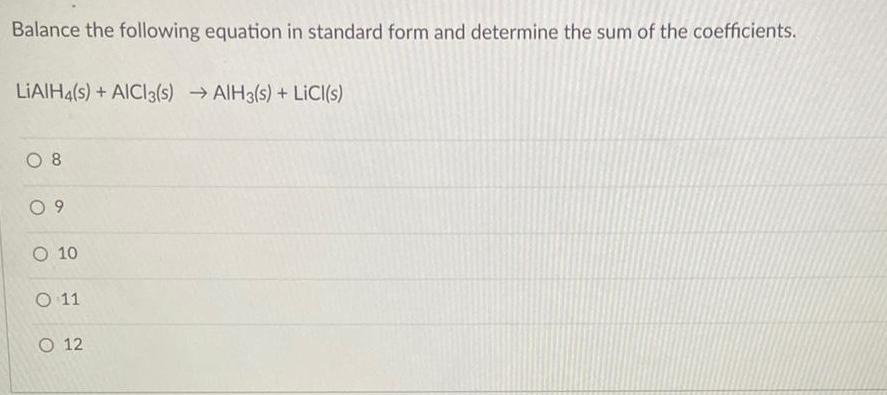Question:

# Balance the following equation in standard form and determine the sum of the coefficients. LIAIH4(s) + AICI3(s) →AIH3(s) + LiCl(s) 08 09 O 10 O 11 O 12

Last updated: 8/8/2022Balance the following equation in standard form and determine the sum of the coefficients. LIAIH4(s) + AICI3(s) →AIH3(s) + LiCl(s) 8 9 10 11 12